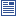Accessibility options:

# Simple applications e.g. finding areas and volumes resources

Show me all resources applicable to

### Community Project (1)Integration for Economics and Business Studies (SOURCE)
Latex source, image files and metadata for the Fact & Formulae leaflet "Integration for Economics and Business Studies " contributed to the mathcentre Community Project by Morgiane Richard (University of Aberdeen) and reviewed by Anthony Cronin (University College Dublin).

### Facts & Formulae Leaflets (1)Integration for Economics and Business Studies
Overview of the rules of integration and their applications in Economics and Business Studies. This leaflet has been contributed to the mathcentre Community Project by Morgiane Richard (University of Aberdeen) and reviewed by Anthony Cronin (University College Dublin).

### Quick Reference (1)Evaluating definite integrals
This leaflet explains what is meant by a definite integral and how it can be evaluated. (Engineering Maths First Aid Kit 8.9)

### Teach Yourself (2)Finding areas by integration
This unit looks at how to calculate the area bounded by a curve using integration.Volumes of solids of revolution
We sometimes need to calculate the volume of a solid which can be obtained by rotating a curve about the x-axis. There is a straightforward technique which enables this to be done, using integration. This unit will explain how.

### Video (2)Finding areas by integration
Integration can be used to calculate areas. In simple cases, the area is given by a single definite integral. But sometimes the integral gives a negative answer which is minus the area, and in more complicated cases the correct answer can be obtained only by splitting the area into several parts and adding or subtracting the appropriate integrals. (Mathtutor Video Tutorial) This resource is released under a Creative Commons license Attribution-Non-Commercial-No Derivative Works and the copyright is held by Skillbank Solutions Ltd.Volumes of solids of revolution
We sometimes need to calculate the volume of a solid which can be obtained by rotating a curve about the x-axis. There is a straightforward technique which enables this to be done, using integration. (Mathtutor Video Tutorial) This resource is released under a Creative Commons license Attribution-Non-Commercial-No Derivative Works and the copyright is held by Skillbank Solutions Ltd.

### Video with captions which require edits (2)Finding areas by integration
Integration can be used to calculate areas. In simple cases, the area is given by a single definite integral. But sometimes the integral gives a negative answer which is minus the area, and in more complicated cases the correct answer can be obtained only by splitting the area into several parts and adding or subtracting the appropriate integrals. (Mathtutor Video Tutorial) The video is released under a Creative Commons license Attribution-Non-Commercial-No Derivative Works and the copyright is held by Skillbank Solutions Ltd.Volumes of solids of revolution
We sometimes need to calculate the volume of a solid which can be obtained by rotating a curve about the x-axis. There is a straightforward technique which enables this to be done, using integration. (Mathtutor Video Tutorial) The video is released under a Creative Commons license Attribution-Non-Commercial-No Derivative Works and the copyright is held by Skillbank Solutions Ltd.